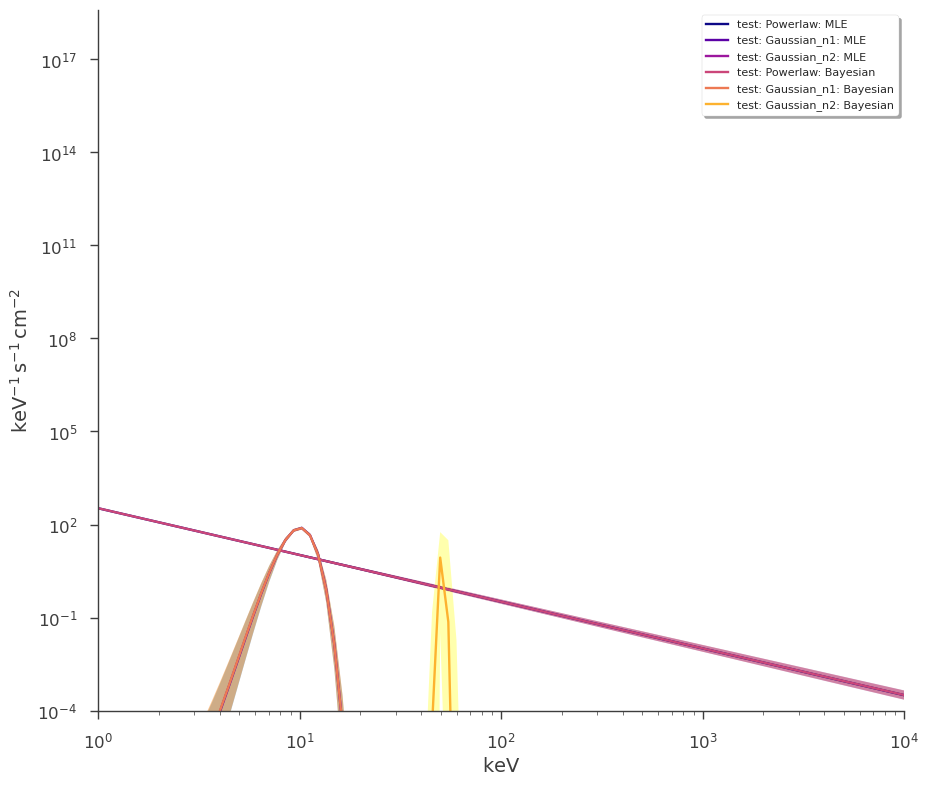# Point source plotting basics

In 3ML, we distinguish between data and model plotting. Data plots contian real data points and the over-plotted model is (sometimes) folded through an instrument response. Therefore, the x-axis is not always in the same units across instruments if there is energy dispersion.

However, all instuments see the same model and a multi-wavelength fit can be viewed in model space without complication. 3ML uses one interface to plot both MLE and Bayesian fitted models. To demonstrate we will use toy data simulated from a powerlaw and two gaussians for MLE fits and an exponentially cutoff power law with one gaussian for Bayesian fits.

First we load the analysis results:

:

import warnings

warnings.simplefilter("ignore")
import numpy as np

np.seterr(all="ignore")

:

{'divide': 'warn', 'over': 'warn', 'under': 'ignore', 'invalid': 'warn'}

:

%%capture
import matplotlib.pyplot as plt

import astropy.units as u
from threeML import *
from threeML.io.package_data import get_path_of_data_file

:

from jupyterthemes import jtplot

%matplotlib inline
jtplot.style(context="talk", fscale=1, ticks=True, grid=False)
silence_warnings()
set_threeML_style()


## Generate some data

:

p = Powerlaw(K=10, piv=10, index=-1.5)

p.K.prior = Log_uniform_prior(lower_bound=1, upper_bound=15)
p.index.prior = Uniform_prior(lower_bound=-3, upper_bound=0)

g1 = Gaussian(F=200, mu=10, sigma=1)
g1.F.prior = Log_uniform_prior(lower_bound=100, upper_bound=300)
g1.mu.prior = Log_uniform_prior(lower_bound=1, upper_bound=50)
g1.sigma.prior = Log_uniform_prior(lower_bound=0.01, upper_bound=10)

g2 = Gaussian(F=200, mu=50, sigma=1)
g2.F.prior = Log_uniform_prior(lower_bound=90, upper_bound=300)
g2.mu.prior = Log_uniform_prior(lower_bound=10, upper_bound=100)
g2.sigma.prior = Log_uniform_prior(lower_bound=0.01, upper_bound=2)

f = p + g1 + g2
x = np.logspace(0, 3, 50)

xy = XYLike.from_function("test", f, x, poisson_data=True)

fig = xy.plot(x_scale="log", y_scale="log")

ps = PointSource("test", 0, 0, spectral_shape=f)
model = Model(ps)
dl = DataList(xy)

08:30:51 INFO      Using Poisson log-likelihood                                                       XYLike.py:112

08:30:53 INFO      Using Poisson log-likelihood                                                       XYLike.py:112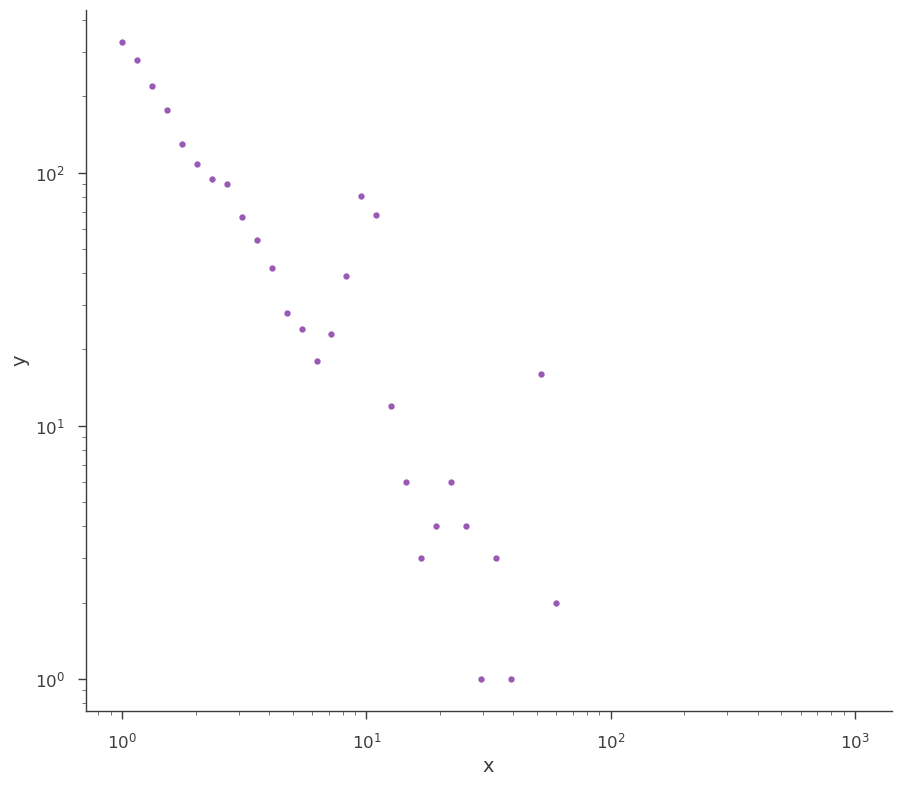:

bayes = BayesianAnalysis(model, dl)
bayes.set_sampler("emcee")
bayes.sampler.setup(n_burn_in=500, n_walkers=100, n_iterations=500)
bayes.sample(quiet=True)
bayes1 = bayes.results

08:30:54 INFO      sampler set to emcee                                                    bayesian_analysis.py:197

08:31:46 INFO      Mean acceptance fraction: 0.24304000000000003                               emcee_sampler.py:157

08:32:00 INFO      fit restored to maximum of posterior                                         sampler_base.py:178

         INFO      fit restored to maximum of posterior                                         sampler_base.py:178

:

jl = JointLikelihood(model, dl)
jl.fit(quiet=True)
mle1 = jl.results

         INFO      set the minimizer to minuit                                             joint_likelihood.py:1042


## Plotting a single analysis result

The easiest way to plot is to call plot_spectra. By default, it plots in photon space with a range of 10-40000 keV evaluated at 100 logrithmic points:

:

_ = plot_spectra(bayes1, ene_min=1, ene_max=1e3, num_ene=300)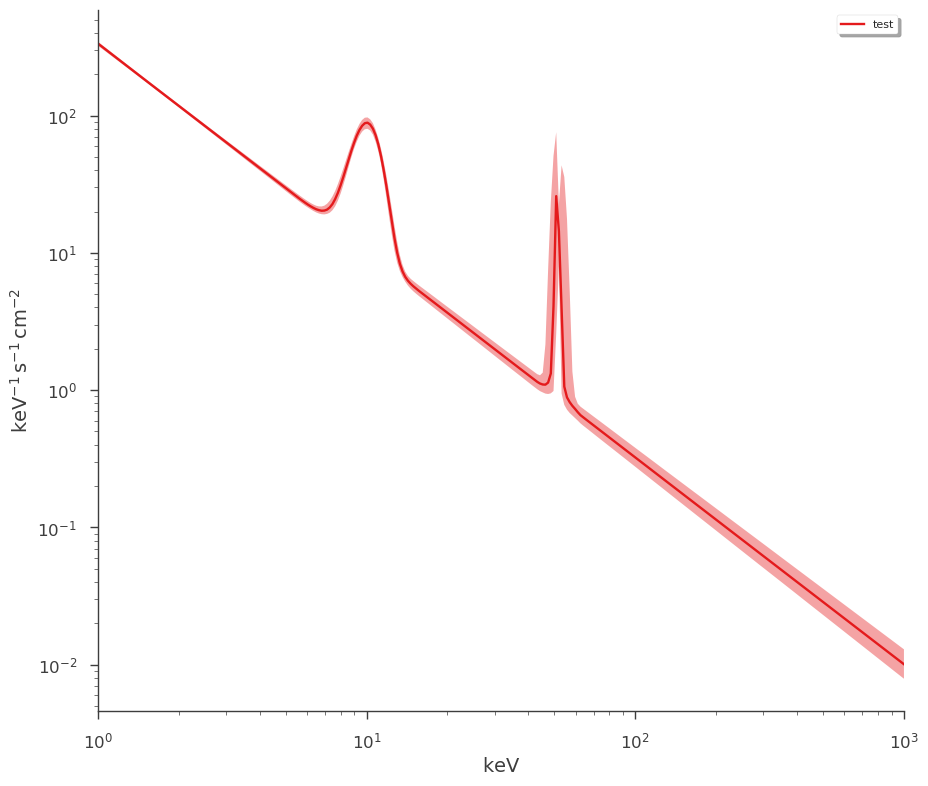### Flux and energy units

We use astropy units to specify both the flux and energy units. * The plotting routine understands photon, energy ($$F_{\nu}$$) and $$\nu F_{ \nu}$$ flux units;

• energy units can be energy, frequency, or wavelength

• a custom range can be applied.

#### changing flux units

:

_ = plot_spectra(mle1, ene_min=1, ene_max=1e3, flux_unit="1/(m2 s MeV)")
_ = plot_spectra(mle1, ene_min=1, ene_max=1e3, flux_unit="erg/(cm2 day keV)")
_ = plot_spectra(mle1, ene_min=1, ene_max=1e3, flux_unit="keV2/(cm2 s keV)")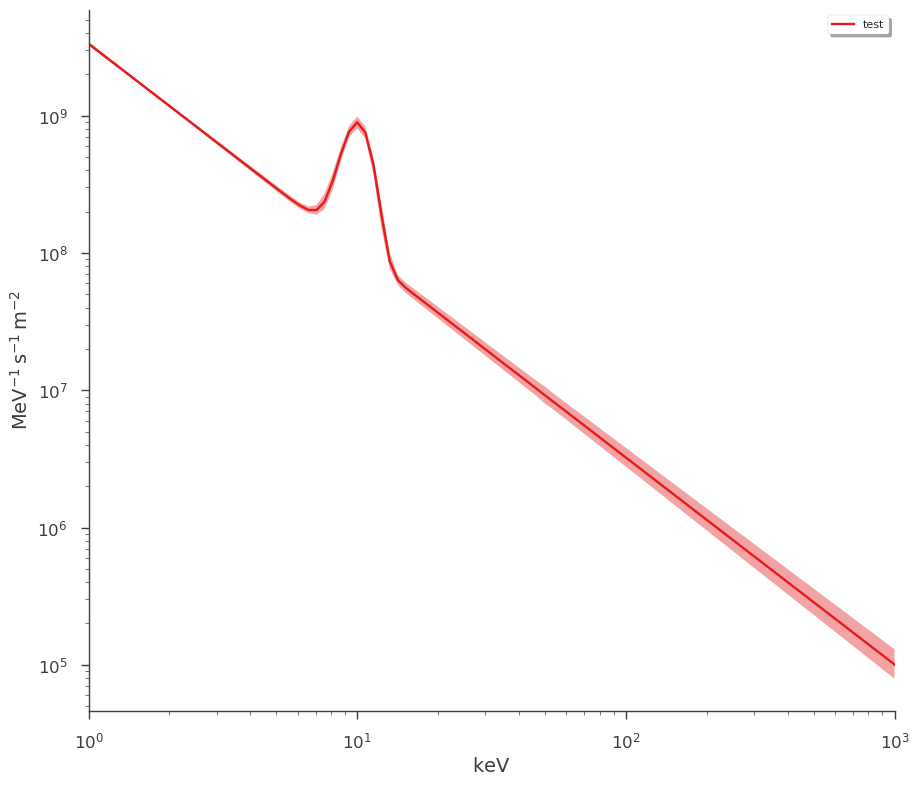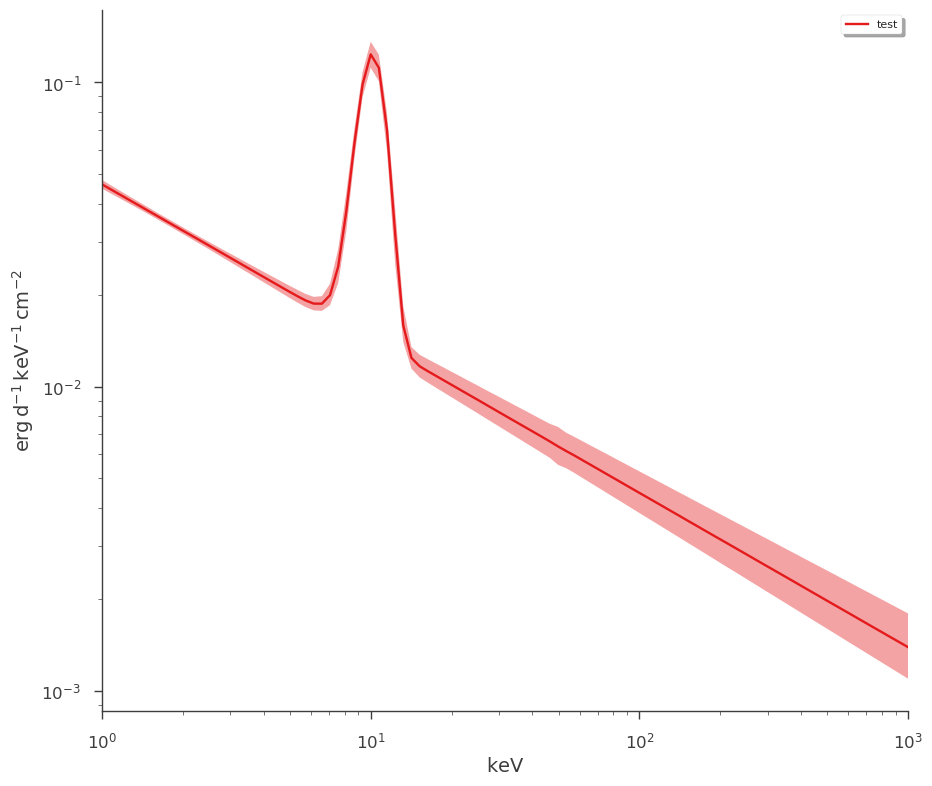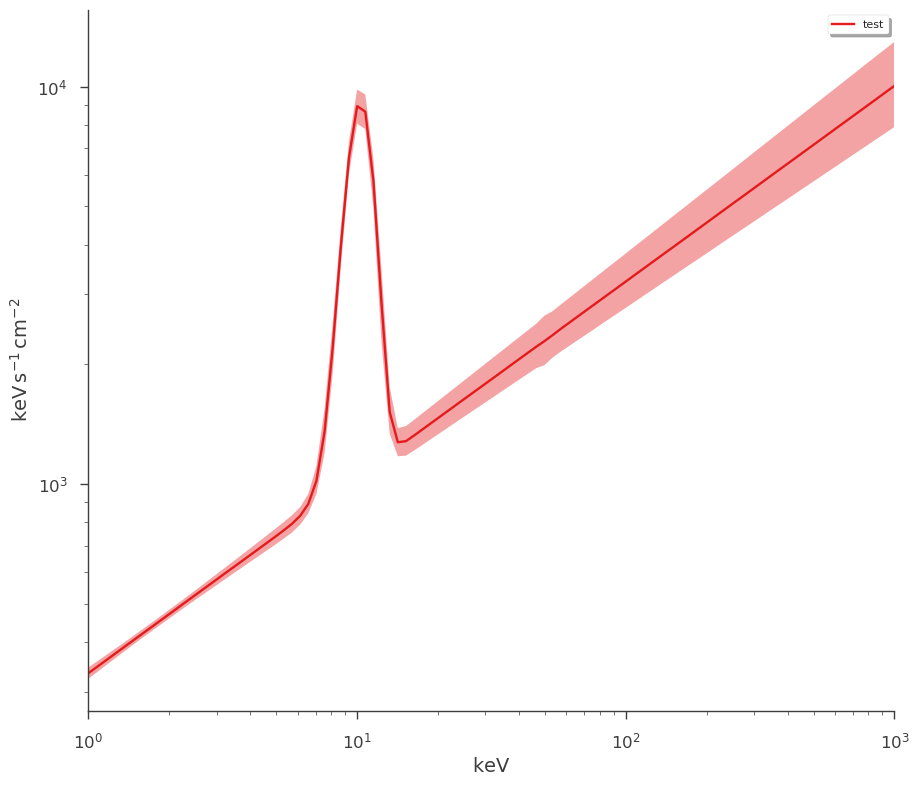#### changing energy units

:

_ = plot_spectra(mle1, ene_min=0.001, ene_max=1e3, energy_unit="MeV")

# energy ranges can also be specified in units
_ = plot_spectra(mle1, ene_min=1 * u.keV, ene_max=1 * u.MeV)

_ = plot_spectra(mle1, ene_min=1e3 * u.Hz, ene_max=1e7 * u.Hz)

_ = plot_spectra(
mle1, ene_min=1e1 * u.nanometer, ene_max=1e3 * u.nanometer, xscale="linear"
)  # plotting with a linear scale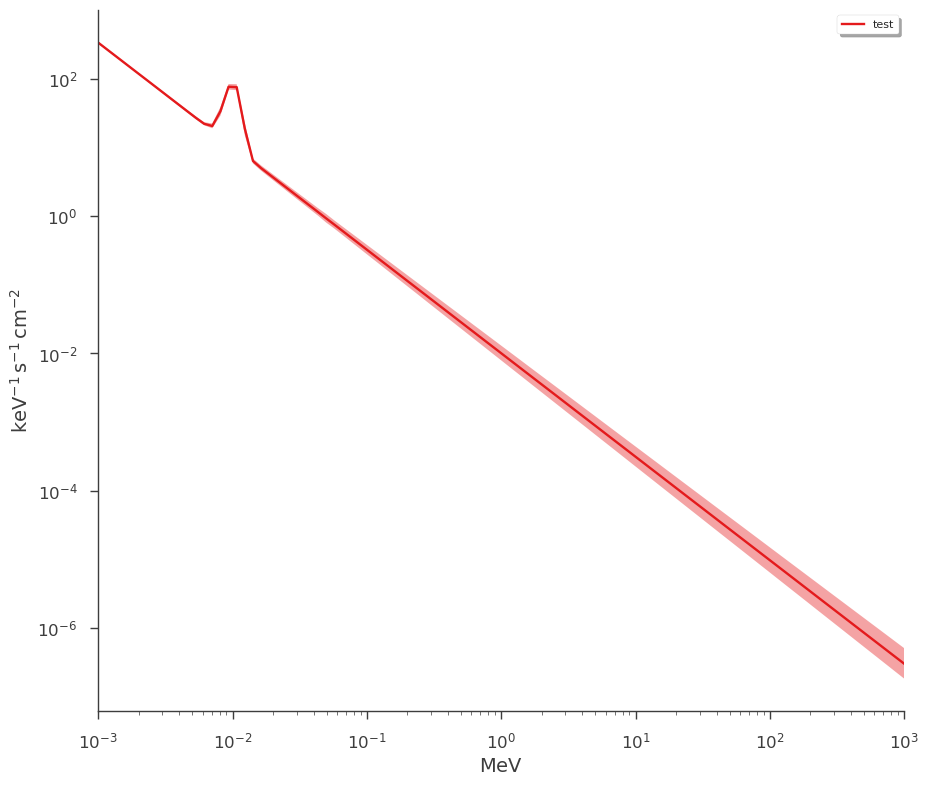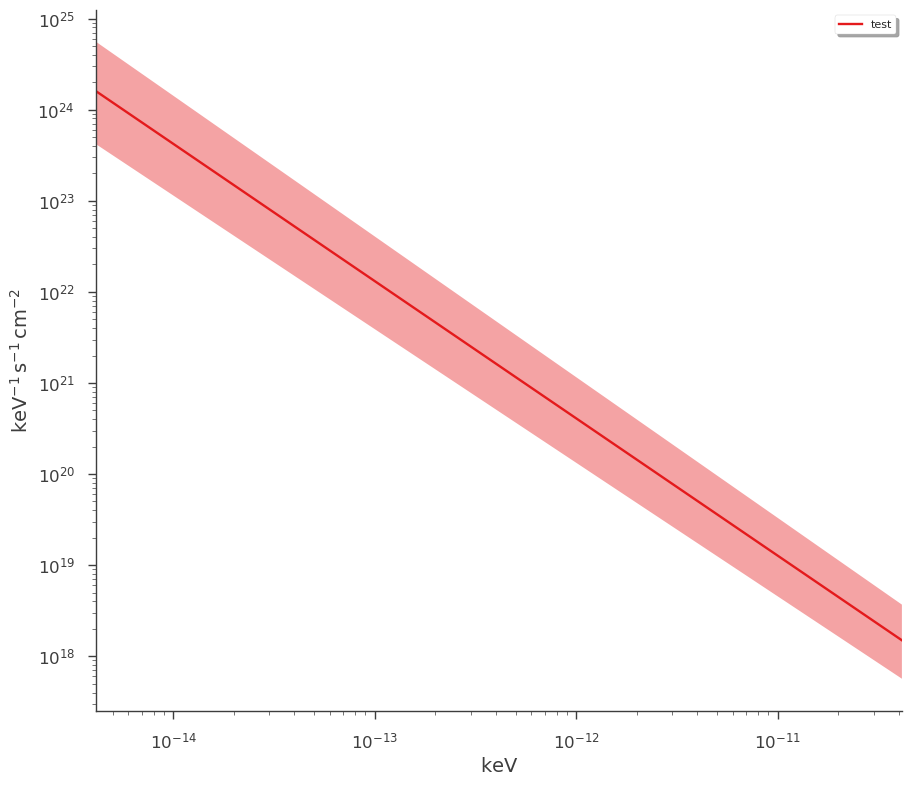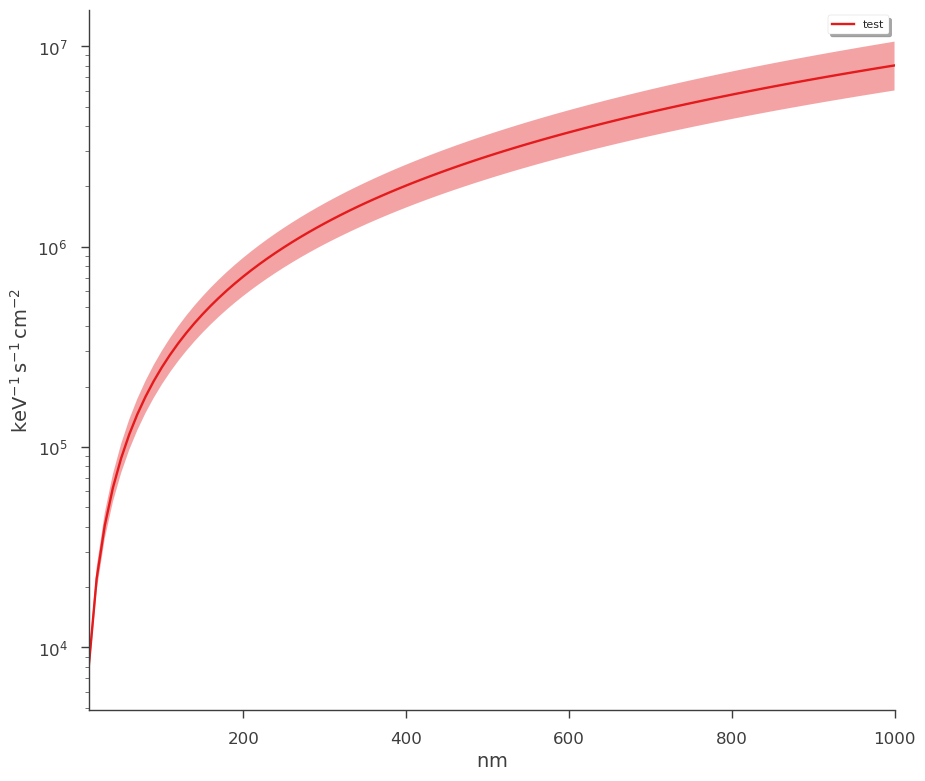### Plotting components

Sometimes it is interesting to see the components in a composite model. We can specify the use_components switch. Here we will use Bayesian results. Note that all features work with MLE of Bayesian results.

:

_ = plot_spectra(bayes1, ene_min=1, ene_max=1e3, use_components=True, num_ene=300)

_ = plt.ylim(bottom=0.1, top=1000)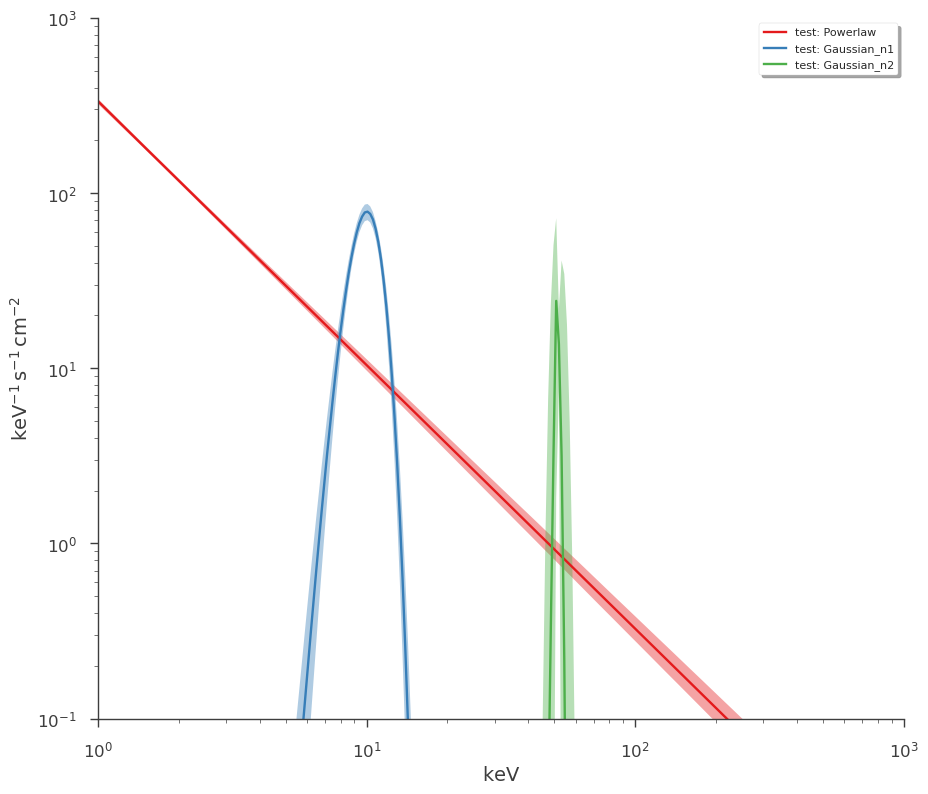Notice that the duplicated components have the subscripts n1 and n2. If we want to specify which components to plot, we must use these subscripts.

:

_ = plot_spectra(
mle1,
flux_unit="erg/(cm2 s keV)",
ene_min=1,
ene_max=1e3,
use_components=True,
components_to_use=["Gaussian_n1", "Gaussian_n2"],
)

_ = plt.ylim(bottom=1e-20)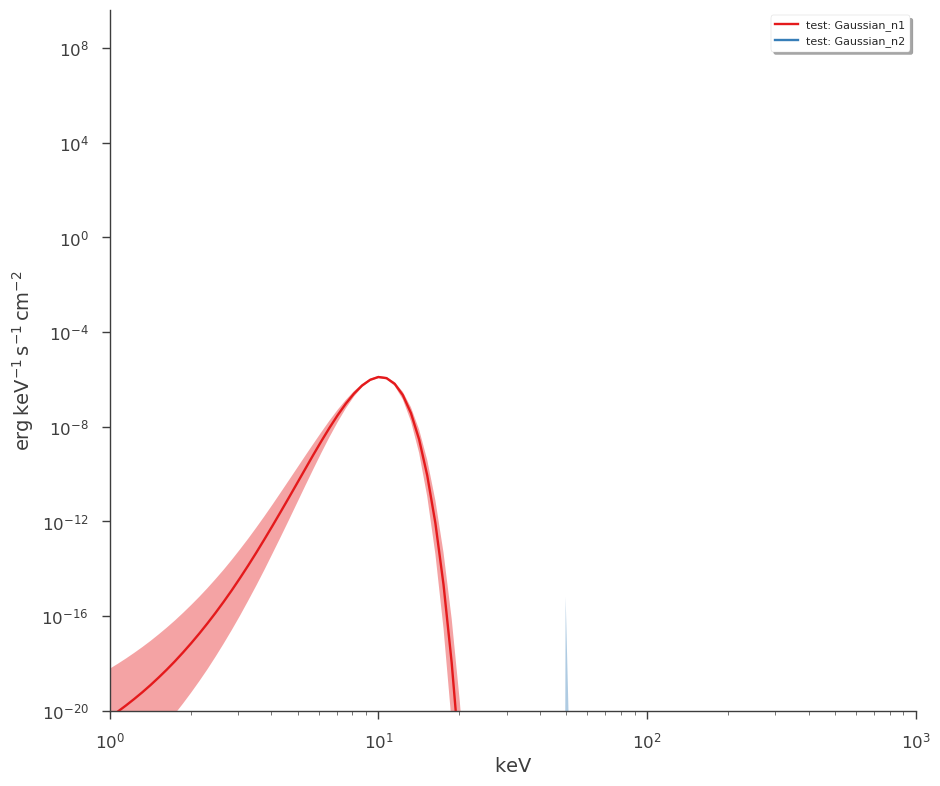If we want to see the total model with the components, just add total to the components list.

Additionally, we can change the confidence interval for the contours from the default of 1$$\sigma$$ (0.68) to 2$$\sigma$$ (0.95).

:

_ = plot_spectra(
bayes1,
flux_unit="erg/(cm2 s keV)",
ene_min=1,
ene_max=1e3,
use_components=True,
components_to_use=["total", "Gaussian"],
confidence_level=0.95,
)

_ = plt.ylim(bottom=1e-9)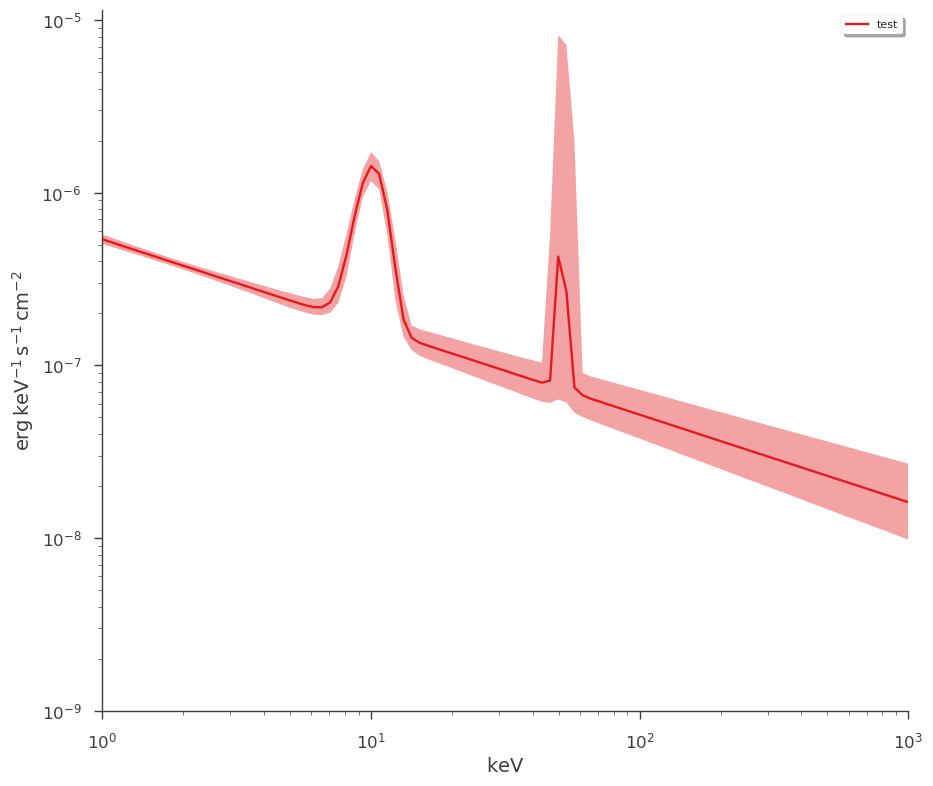:

_ = plot_spectra(
mle1,
flux_unit="erg/(cm2 s keV)",
ene_min=1,
ene_max=1e3,
use_components=True,
fit_cmap="jet",  # specify a color map
contour_colors="k",  # specify a color for all contours
components_to_use=["total", "Gaussian_n2", "Gaussian_n1"],
)

_ = plt.ylim(bottom=1e-16)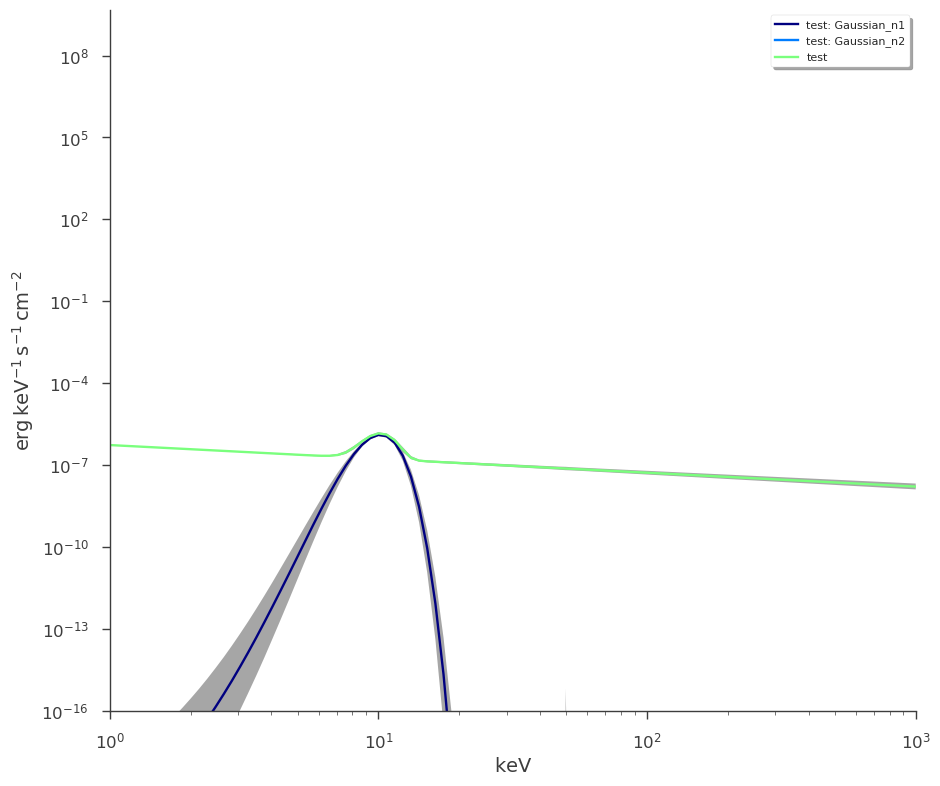Explore the docstring to see all the available options. Default configurations can be altered in the 3ML config file.

• Use asymmetric errors and alter the default color map

:

threeML_config["model_plot"]["point_source_plot"]["fit_cmap"] = "plasma"
_ = plot_spectra(mle1, equal_tailed=False)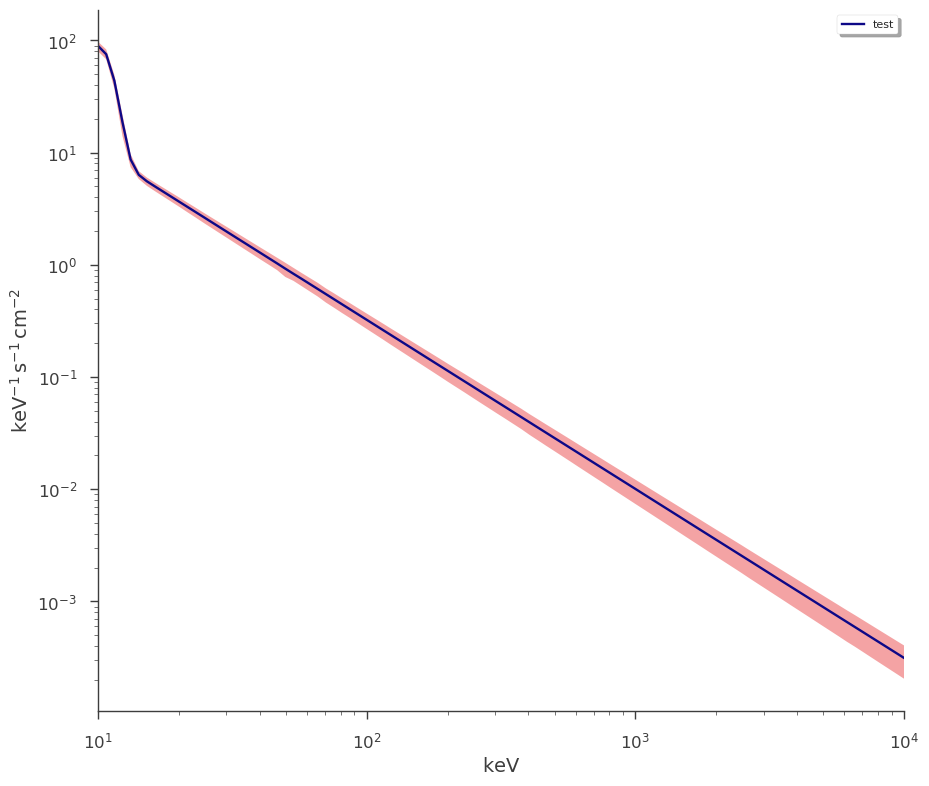• turn of contours and the legend and increase the number of points plotted

:

_ = plot_spectra(mle1, show_legend=False, show_contours=False, num_ene=500)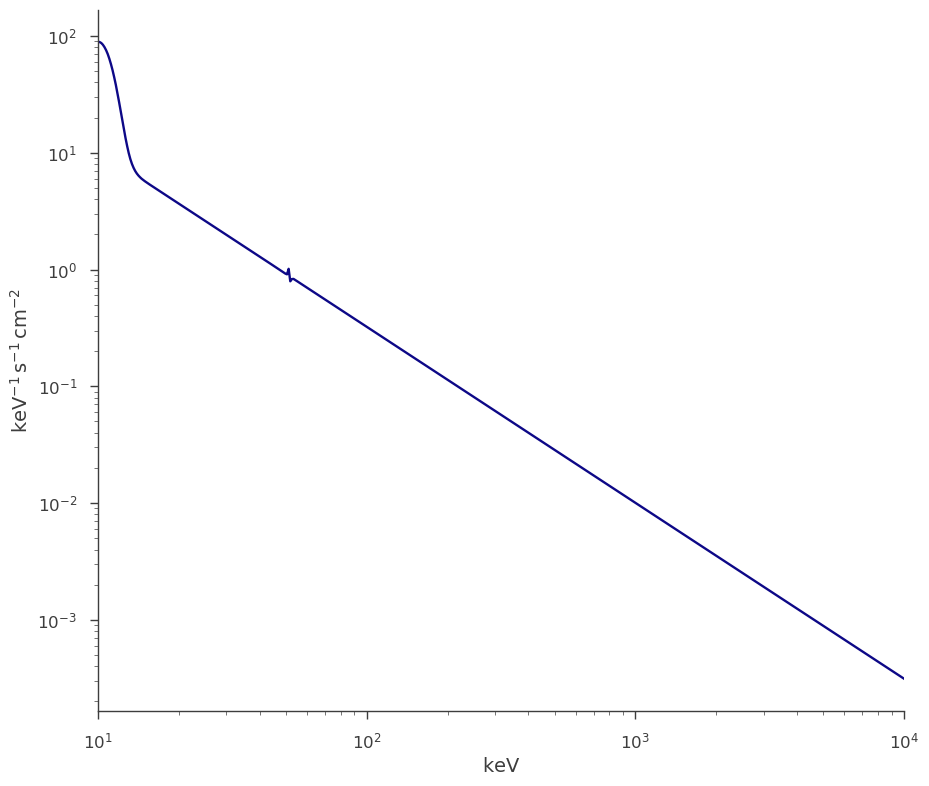• colors or color maps can be specfied

:

_ = plot_spectra(mle1, fit_colors="orange", contour_colors="blue")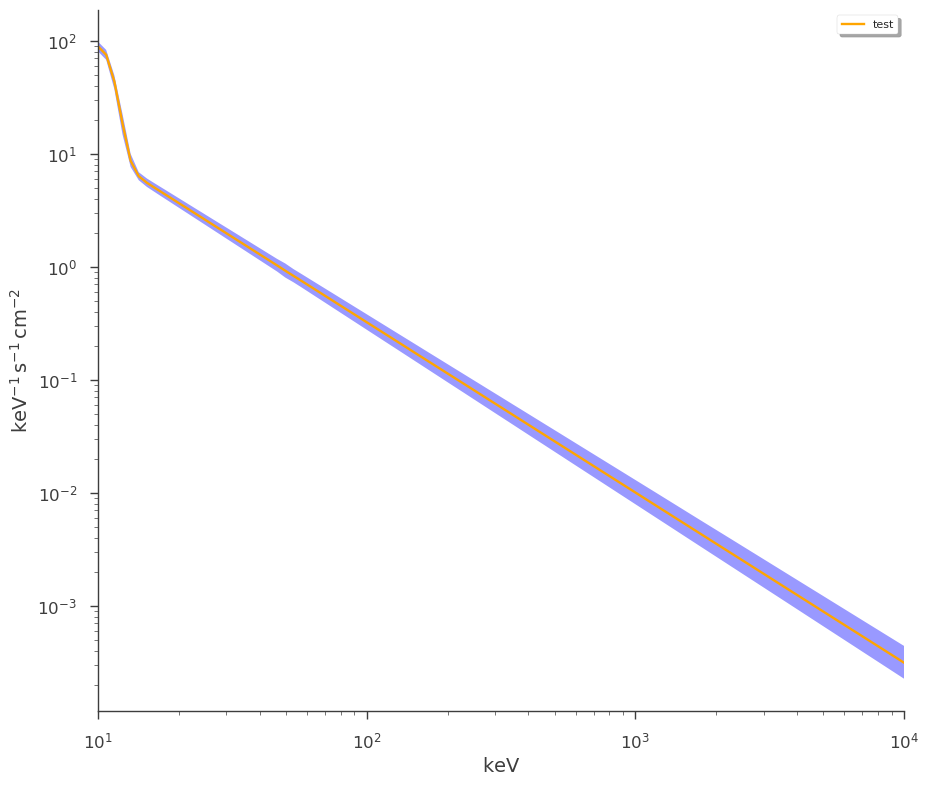Further modifications to plotting style, legend style, etc. can be modified either in the 3ML configuration:

:

show_configuration("model_plot")

:

config
┗━━ point_source_plot
┣━━ fit_cmap: MPLCmap.plasma
┣━━ contour_cmap: MPLCmap.Set1
┣━━ bayes_cmap: MPLCmap.Set1
┣━━ plot_style
┃   ┣━━ linestyle: -
┃   ┗━━ linewidth: 1.7
┣━━ contour_style
┃   ┗━━ alpha: 0.4
┣━━ show_legend: True
┣━━ legend_style
┃   ┣━━ loc: best
┃   ┣━━ fancybox: True
┣━━ flux_unit: 1/(keV s cm2)
┣━━ emin: 10.0
┣━━ emax: 10000.0
┣━━ num_ene: 100
┗━━ ene_unit: keV


or by directly passing dictionary arguments to the the plot command. Examine the docstring for more details!

## Plotting multiple results

Any number of results can be plotted together. Simply provide them as arguments. You can mix and match MLE and Bayesian results as well as plotting their components.

:

_ = plot_spectra(mle1, bayes1, ene_min=1)

# _=plt.ylim(bottom=1E-1)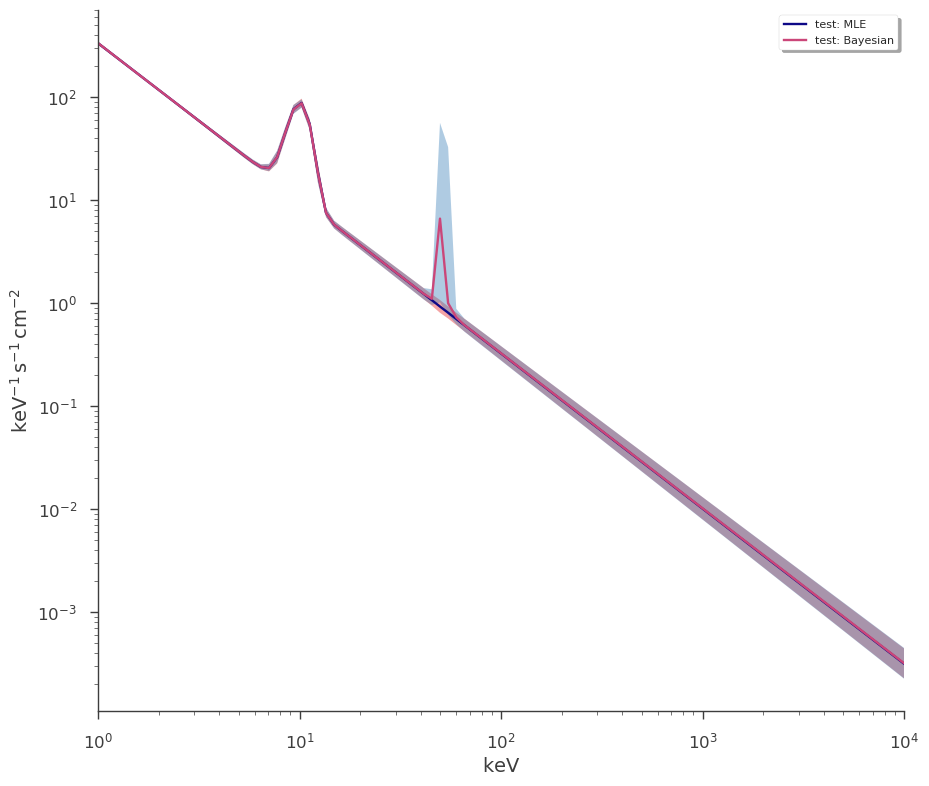Specify particular colors for each analysis and broaden the contours

:

_ = plot_spectra(
mle1,
bayes1,
ene_min=1.0,
confidence_level=0.95,
equal_tailed=False,
fit_colors=["orange", "green"],
contour_colors="blue",
)
_ = plt.ylim(bottom=1e-1)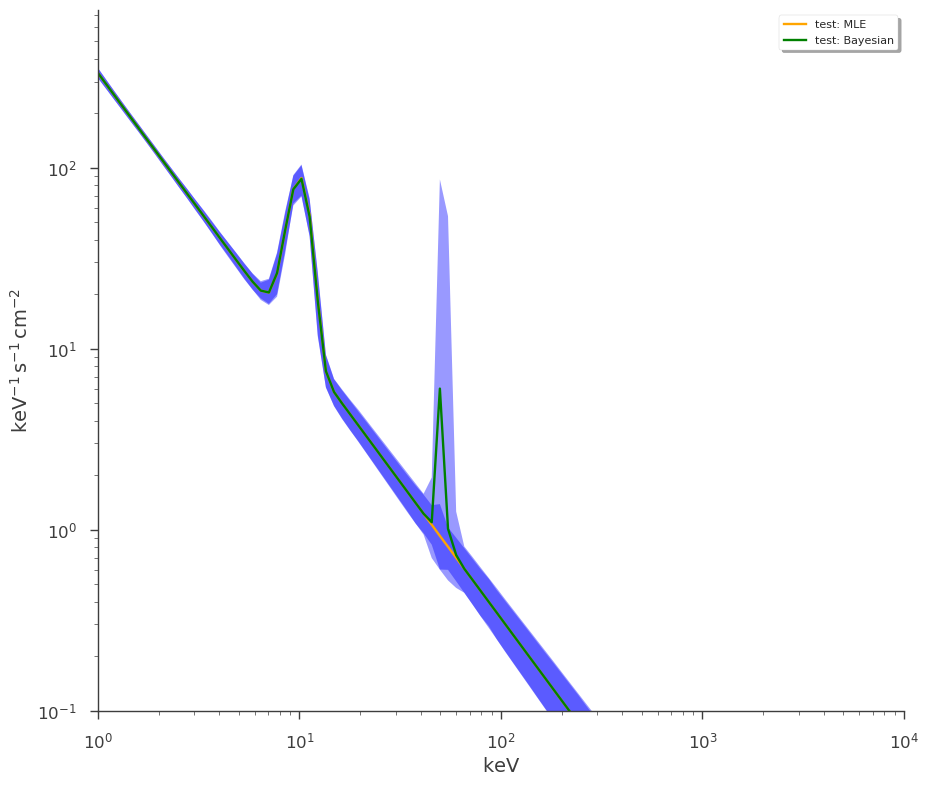As with single results, we can choose to plot the components for all the sources.

:

_ = plot_spectra(mle1, bayes1, ene_min=1.0, use_components=True)
_ = plt.ylim(bottom=1e-4)